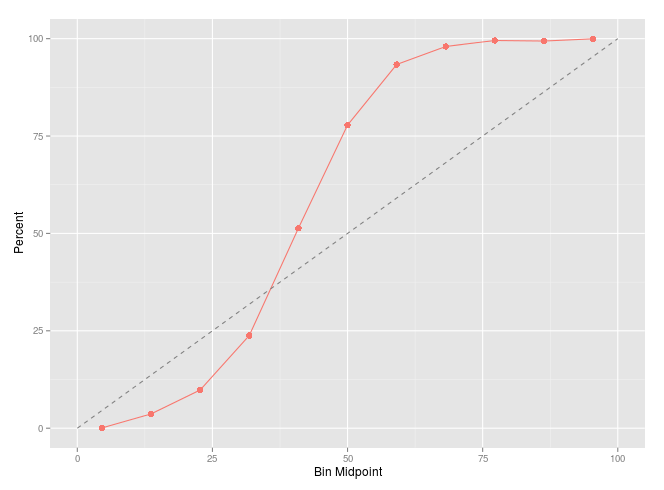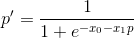# Model Calibration

The performance of a trained classification model can be measured in several ways. Accuracy is one important aspect that is typically the major driver while developing predictive models. Accuracy is what usually tells you which model to choose. However, the predictions of a good model should not only be accurate, but also well-calibrated.

To measure this quality, a calibration plot can show the relation between the true class of the samples and the predicted probabilities. This will give a measure of how realistic a model prediction is. For example, if in reality an event occurs 3 out of 10 times, a realistic model will yield predictions with an event rate of about 30%.

Given a set of samples with their true outcomes known, we can predict their class probabilities using a pre-trained model. To observe how well these predictions are calibrated, we bin the samples according to their class probabilities generated by the model. You could experiment with different binnings, but a commonly used binning is: [0,10%], (10,20%], (20,30%], … (90,100%]. The following step is to identify the event rate of each bin. For example, if 4 out of 5 samples falling into the last bin are actual events (their true outcome belongs to the class of interest), then the event rate for that bin would be 80%. The calibration plot displays the bin mid-points on the x-axis and the event rate on the y-axis. Ideally, the event rate should start very low with first bin and gradually increase until the last bin, which would be reflected as a 45◦ line.

To walk through an example, let us see how we can apply this in R. The data used here is from an ongoing Kaggle competition (at the time of this writing). This is a two-class problem of predicting whether an insurance quote will be purchased by a given customer. Based on the Receiver Operating Characteristic (ROC) curve, the accuracy metric used here is the Area Under the Curve (AUC), similar to other Kaggle competitions.

First we read in the data which we will use for testing:

`library(readr)test = read_csv("./input/data.csv")id_name = "QuoteNumber"target_name = "QuoteConversion_Flag"`

Predict the data using our pre-trained model:

`library(xgboost)library(caret)library(ggplot)model = xgb.load("./models/model1.xgb")# excluding id and target columnspredict_matrix = data.matrix(test[, -which(                   names(test) %in% c(target_name, id_name))])probs = predict(model, predict_matrix)class_probs = data.frame(  target = factor(test[[target_name]]),  prediction = probs)`

Now that we have our class probabilities and the true classes in one data.frame, we can draw a calibration plot like this:

`cal_plot_data = calibration(target ~ prediction,   data = class_probs, class = 1)\$dataggplot() + xlab("Bin Midpoint") +  geom_line(data = cal_plot_data, aes(midpoint, Percent),            color = "#F8766D") +  geom_point(data = cal_plot_data, aes(midpoint, Percent),            color = "#F8766D", size = 3) +  geom_line(aes(c(0, 100), c(0, 100)), linetype = 2,             color = 'grey50')`A calibration plot for the class probabilities predicted by a pre-trained model

It turns out that the model’s calibration plot is not as close to a 45◦ line as we would like. We should, therefore, try to improve the calibration of this model’s predictions. Note how the plot follows a sigmoidal pattern. If you are familiar with the formula for logistic regression, you may expect the next step.p’: the calibrated class probability as a function of the un-calibrated class probability p

One way to calibrate these probabilities is to train a logistic regression model (coincidence?) to predict the true class of a sample as a function of the its un-calibrated class probability.

`train = read_csv("./input/train.csv")lr_model = glm(target ~ prediction, data = train,  family = binomial)coef(summary(lr_model))#              Estimate Std. Error   z value Pr(>|z|)# (Intercept) -5.109892 0.07319075 -69.81609        0# prediction  12.486464 0.20758641  60.15068        0`

Now we can use the logistic regression model to predict the calibrated class probabilities:

`lr_probs = predict(lr_model,   newdata = class_probs[, 'prediction', drop = FALSE],  type = "response")class_lr_probs = data.frame(  target = factor(test[[target_name]]),  prediction = lr_probs)cal_lr_plot_data = calibration(target ~ prediction,                            data = class_lr_probs, class = 1)\$data`

Let’s gather both calibrations in one data.frame for plotting:

`library(tidyr)library(dplyr)plot_data = cal_plot_data %>%   mutate("Un-calibrated" = Percent,         "Calibrated" = cal_lr_plot_data[, "Percent"]) %>%   select(-Percent) %>%   gather_("Stage", "Percent", c("Un-calibrated", "Calibrated"))ggplot() + xlab("Bin Midpoint") +  geom_line(data = plot_data, aes(midpoint, Percent, fill = Stage, color = Stage)) +  geom_point(data = plot_data, aes(midpoint, Percent, fill = Stage, color = Stage), size = 3) +  geom_line(aes(c(0, 100), c(0, 100)), linetype = 2,             color = 'grey50')`

Et, voila!

The calibrated class probabilities follow the 45◦ line a lot more closely than the un-calibrated. This implies that the event rate for predictions is more realistic, which gives us a two-stage model framework. In the first stage we use our training set to generate initial class probabilities, then in the second stage we calibrate these probabilities as a function of the true outcome.

For further reading about model calibration, check out these resources:

Thanks to my colleague Yusuf Saber for proof reading and offering valuable suggestions.

How this post came to be? Every week at Optima, everyone on the team gets five minutes or so to share a “nugget” of data science, algorithms or related knowledge. The only rule is that it can be explained and grasped in 5 to 10 minutes. Lately we decided to share these nuggets with the world. So here we are.

--

--

## More from Optima . Blog

Thoughts on data, technology, startups, and oftentimes, other things.